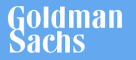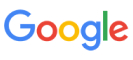New update is available. Click here to update.
Topics

# Decode String

Easy0/40
Average time to solve is 15m+8 more companies

## Problem statement

You have been given an encoded string. Your task is to decode it back to the original string.

``````- An encoded string will be of the form <count>[encoded_string], where the 'encoded_string' inside the square brackets is being repeated exactly 'count' times. Note that 'count' is guaranteed to be a positive integer and can be greater than 9.
- There are no extra white spaces and square brackets are well-formed.
``````
For example -
``````Input: 2[a]
“a” is encoded 2 times, hence the decoded string will be "aa".

Input: 3[a2[b]]
“b” is encoded 2 times, which decodes as 3[abb]. Now, "abb" is encoded 3 times, hence decoded string will be "abbabbabb".
``````
Detailed explanation ( Input/output format, Notes, Images )
Constraints:
`````` 1 <= T <= 20
1 <= |S| <= 500

where |S| is the length of the Decoded string.

Time limit: 0.400 sec
``````
Sample Input 1:
``````2
3[a]2[bc]
a1[b]1[cc]
``````
Sample Output 1:
``````aaabcbc
abcc
``````
Explanation for sample 1:
``````For the first test case, "a" is encoded 3 times and "bc" is encoded 2 times. After combining these two strings after decoding, we'll get "aaa" + "bcbc" = "aaabcbc".
``````
Sample Input 2:
``````1
ab2[c3[b]]
``````
Sample Output 2:
``````abcbbbcbbb
``````Console